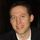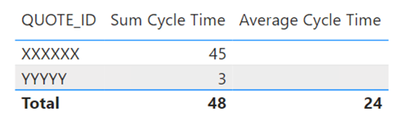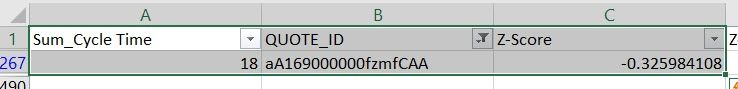cancel
Showing results for
Did you mean:Anonymous
Not applicable

## Average on a calculated sum

I have done a fair amount of searching in the community and am not finding what I am looking for and am hoping someone can help. Here is my data set:

Cycle Time      QUOTE_ID       Sum       Average for all
0                      XXXXXX
1                       XXXXXX
4                       XXXXXX
18                      XXXXXX
22                        XXXXXX       45
1                           YYYYY
2                          YYYYY            3
48                    24

I have calculated the aggregated sum for each of my Quote IDs as: SUMX(VALUES([QUOTE_ID]),CALCULATE(SUM([Cycle Time]))). I am trying to get an average that goes across the aggregated sum by quote (and will include filters on the dashboard for geography, etc.). In the example above, the two amounts that are summed for the quotes are 48 so the average would be 24.  I can't get the correct average amount for the aggregated sum.

2 ACCEPTED SOLUTIONSSuper User

@Anonymous,

Try these measures:

``````Sum Cycle Time =
SUMX ( VALUES ( Table1[QUOTE_ID] ), CALCULATE ( SUM ( Table1[Cycle Time] ) ) )``````

``````Average Cycle Time =
VAR vAvg =
AVERAGEX ( VALUES ( Table1[QUOTE_ID] ), [Sum Cycle Time] )
VAR vResult =
IF ( NOT HASONEVALUE ( Table1[QUOTE_ID] ), vAvg )
RETURN
vResult``````Proud to be a Super User!Super User

@Anonymous,

Try these measures:

``````Sum Cycle Time = SUM ('CPQ Negotiating'[Cycle Time] )

Mean =
CALCULATE (
AVERAGEX ( VALUES ( 'CPQ Negotiating'[QUOTE_ID] ), [Sum Cycle Time] ),
ALLSELECTED ( 'CPQ Negotiating'[QUOTE_ID] )
)

Std Dev =
CALCULATE (
STDEVX.P ( VALUES ( 'CPQ Negotiating'[QUOTE_ID] ), [Sum Cycle Time] ),
ALLSELECTED ( 'CPQ Negotiating'[QUOTE_ID] )
)

Z-Score = DIVIDE ( [Sum Cycle Time] - [Mean], [Std Dev] )``````I manually entered the first two QUOTE_ID, so Mean, Std Dev, and Z-Score are different from yours. Let me know the result with your full data set.

Proud to be a Super User!

6 REPLIES 6Super User

@Anonymous,

Try these measures:

``````Sum Cycle Time =
SUMX ( VALUES ( Table1[QUOTE_ID] ), CALCULATE ( SUM ( Table1[Cycle Time] ) ) )``````

``````Average Cycle Time =
VAR vAvg =
AVERAGEX ( VALUES ( Table1[QUOTE_ID] ), [Sum Cycle Time] )
VAR vResult =
IF ( NOT HASONEVALUE ( Table1[QUOTE_ID] ), vAvg )
RETURN
vResult``````Proud to be a Super User!Anonymous
Not applicable

I am hoping that you can also help me with a standard deviation, ultimately what I'm trying to do is get a z-score for every quote based on the sum of its cycle time against the average based on the sums of the cycle times across the data set. I'm trying something like:

sd =
VAR _CycleTime = 'CPQ Negotiating'[Sum of Cycle Time___]
VAR _AverageCycleTime = 'CPQ Negotiating'[Average Cycle Time__]
VAR _sd
= STDEV.P ('CPQ Negotiating'[Sum of Cycle Time___])
RETURN
([_CycleTime] - [_AverageCycleTime])/_sd

The problem that I am having is when trying to pass the sum of the quote to the standard deviation function.Super User

@Anonymous,

Would you be able to provide the expected result, along with the calculation logic?

Proud to be a Super User!Anonymous
Not applicable

Ultimately what I am trying to do is a Z-score based on the summed values so:

ZScore = ([Sum of Cycle Time]-[Mean])/[Standard Deviation]

Here is an example of what it looks like without the sum (it is going against the individual values which I don't want):
Here is what the Zscore calculation looks like against the raw data (without cycle time summed):Raw data

This is what I am trying to do:So the mean is based on the average of the summed amounts, the standard deviation also goes against the sum of the cycle time.Super User

@Anonymous,

Try these measures:

``````Sum Cycle Time = SUM ('CPQ Negotiating'[Cycle Time] )

Mean =
CALCULATE (
AVERAGEX ( VALUES ( 'CPQ Negotiating'[QUOTE_ID] ), [Sum Cycle Time] ),
ALLSELECTED ( 'CPQ Negotiating'[QUOTE_ID] )
)

Std Dev =
CALCULATE (
STDEVX.P ( VALUES ( 'CPQ Negotiating'[QUOTE_ID] ), [Sum Cycle Time] ),
ALLSELECTED ( 'CPQ Negotiating'[QUOTE_ID] )
)

Z-Score = DIVIDE ( [Sum Cycle Time] - [Mean], [Std Dev] )``````I manually entered the first two QUOTE_ID, so Mean, Std Dev, and Z-Score are different from yours. Let me know the result with your full data set.

Proud to be a Super User!Anonymous
Not applicable

Thank you so much for your help. I had to modify the ALLSELECTED to include some additional columns that are included within the data set but this worked perfectly.Announcements#### Exclusive opportunity for Women!

Join us for a free, hands-on Microsoft workshop led by women trainers for women where you will learn how to build a Dashboard in a Day!#### Power Platform Conference-Power BI and Fabric Sessions

Join us Oct 1 - 6 in Las Vegas for the Microsoft Power Platform Conference.Top Solution Authors
Top Kudoed Authors
Users online (1,907)